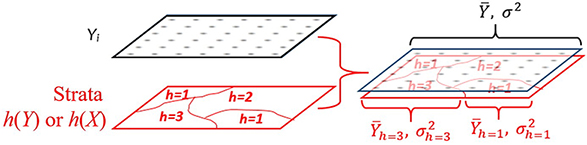# Geodetector method

Spatial stratified heterogeneity (SSH), referring to the within strata are more similar than the between strata, such as landuse types and climate zones, is ubiquitous in spatial data. SSH instead of random is a set of information, which has been being a window for humans to understand the nature since Aristotle time. In another aspect, a model with global parameters would be confounded if input data is SSH, the problem dissolves if SSH is identified so simple models can be applied to each stratum separately. Note that the “spatial” here can be either geospatial or the space in mathematical meaning.

Geodetector is a novel tool to investigate SSH: (1) measure and find SSH of a variable Y ; (2) test the power of determinant X of a dependent variable Y according to the consistency between their spatial distributions; and (3) investigate the interaction between two explanatory variables X1 and X2 to a dependent variable Y. All of the tasks are implementable by the geographical detector q-statistic: $\begin{equation} q=1- \frac{1}{N\sigma^2}\sum_{h=1}^{L}N_h\sigma_h^2 \end{equation}$

where N and σ2 stand for the number of units and the variance of Y in study area, respectively; the population Y is composed of L strata (h = 1, 2, …, L), Nh and σh2 stand for the number of units and the variance of Y in stratum h, respectively. The strata of Y (red polygons in Figure 1) are a partition of Y, either by itself ( h(Y) in Figure 1) or by an explanatory variable X which is a categorical variable ( h(Y) in Figure 1). X should be stratified if it is a numerical variable, the number of strata L might be 2-10 or more, according to prior knowledge or a classification algorithm.Figure 1. Principle of geodetector

(Notation: Yi stands for the value of a variable Y at a sample unit i ; h(Y) represents a partition of Y ; h(X) represents a partition of an explanatory variable X. In geodetector, the terms “stratification”, “classification” and “partition” are equivalent.)

Interpretation of q value (please refer to Fig.1). The value of q ∈ [0, 1].

If Y is stratified by itself h(Y), then q = 0 indicates that Y is not SSH; q = 1 indicates that Y is SSH perfectly; the value of q indicates that the degree of SSH of Y is q.

If Y is stratified by an explanatory variable h(X), then q = 0 indicates that there is no association between Y and X ; q = 1 indicates that Y is completely determined by X ; the value of q-statistic indicates that X explains 100q% of Y. Please notice that the q-statistic measures the association between X and Y, both linearly and nonlinearly.

For more detail of Geodetector method, please refer:

 Wang JF, Li XH, Christakos G, Liao YL, Zhang T, Gu X, Zheng XY. Geographical detectors-based health risk assessment and its application in the neural tube defects study of the Heshun Region, China. International Journal of Geographical Information Science, 2010, 24(1): 107-127.

 Wang JF, Zhang TL, Fu BJ. A measure of spatial stratified heterogeneity. Ecological Indicators,2016, 67(2016): 250-256.

 Wang JF, Xu CD. Geodetector:Principle and prospective. Geographica Sinica,2017,72(1):116-134.

# R package for geodetector

geodetector package includes five functions: factor_detector, interaction_detector, risk_detector, ecological_detector and geodetector. The first four functions implementing the calcution of factor detector, interaction detector, risk detector and ecological detector, which can be calculated using table data, e.g. csv format(Table 1). The last function geodetector is an auxiliary function, which can be used to implement the calculation for shapefile format map data(Figure 2).

Table 1. Demo data in table format
incidence watershed soiltype elevation
7.20 2 3 6
7.01 2 3 6
6.79 2 3 6
6.73 4 3 6
6.77 4 3 1
6.74 4 3 6

geodetector package depends on the following packages: rgeo, sp, maptools and rgdal, which should be installed in advance.

As a demo, neural-tube birth defects (NTD) Y and suspected risk factors or their proxies Xs in villages are provided, including data for the health effect GIS layers and environmental factor GIS layers, “elevation”, “soil type”, and “watershed”.